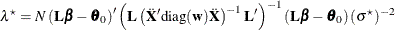# The GLMPOWER Procedure

If you specify covariates in the model (whether continuous or categorical), then two adjustments are made in order to compute approximate power in the presence of the covariates. Let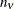denote the number of covariates (counting dummy variables for categorical covariates individually). In other words,is the total degrees of freedom used by the covariates. The adjustments are as follows:

1. The error degrees of freedom decrease by.

2. The error standard deviationshrinks by a factor of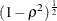(if the CORRXY= option to specify the correlation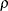between covariates and response) or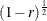(if the PROPVARREDUCTION= option is used to specify the proportional reduction in total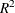incurred by the covariates). Let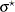represent the updated value of.

As a result of these changes, the power is computed as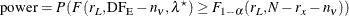whereis calculated usingrather than: